# Fibonacci method

(diff) ← Older revision | Latest revision (diff) | Newer revision → (diff)

A variety of processes of one-dimensional search for an extremum of a function by means of successively narrowing the interval of uncertainty. The sole restriction imposed on the function in question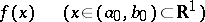is the requirement of strict unimodality on the given interval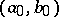.

In the successive narrowing process the values ofare computed (or measured) at a numberof test points that is bounded beforehand. As a result one obtains a sequence of narrowing intervals of uncertainty containing the required extremum:In order to narrow the interval of uncertainty for an arbitrary strictly-unimodal function, one needs to know not less than two test values of it. In the Fibonacci method one chooses exactly two test values inside each current interval of uncertainty, symmetrically about the middle of the interval. Next, along with one of the test points one discards the end of the interval with the worst values of. One obtains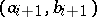, where in addition to the remaining old test point one symmetrically constructs a new one. Hence for the sequence of intervalsfollows the recurrence relation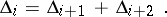(It is also assumed above that the overlap conditionis satisfied.)

The solution to the equation under the conditiongiveswhere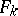are the Fibonacci numbers.

For the extremum point one finds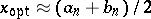.

In the simplest version of the Fibonacci method (when one assumes that the test points and test valuesare determined absolutely accurately), in order to narrow the original interval of uncertainty fromtoone needs to take a numberof test points satisfying the inequality. Taking into account accuracy corrections to the determination of the values of the function, the above estimate becomes somewhat more complicated.

The limit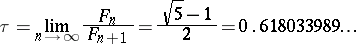exists and gives the reason for introducing the golden section method — a version of the Fibonacci method such that for all,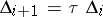, that is, the test points make a golden section of the current interval (cf. also Golden ratio). An advantage of this method consists in the fact that the number of test points does not have to be planned in advance.

Modifications of the Fibonacci method have been developed for functions of non-compact support, for shortening the calculations whenis equal at two test points, for increasing the stability of the calculation, etc.

The Fibonacci method is significantly more efficient than that of dichotomy (see Dichotomy method). But for optimizing differentiable functions other methods are preferable (see Descent, method of; Maximization and minimization of functions).

How to Cite This Entry:
Fibonacci method. Encyclopedia of Mathematics. URL: http://encyclopediaofmath.org/index.php?title=Fibonacci_method&oldid=13380
This article was adapted from an original article by Yu.P. IvanilovV.V. Okhrimenko (originator), which appeared in Encyclopedia of Mathematics - ISBN 1402006098. See original article